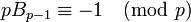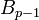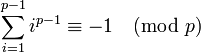# Agoh-Giuga conjecture

## Statement

### Formulation in terms of Bernoulli numbers

This formulation dates to Agoh in 1990. It states that a natural numberis a prime number if and only if we have:whereis the Bernoulli number corresponding to.

### Formulation in terms of power sums

This formulation dates to Giuga in the 1950s.

A natural numberis a prime number if and only if we have:Note that one direction is immediate: ifis a prime number, then the above congruence holds true as a consequence of Fermat's little theorem. The other direction is conjectural and open.

### Formulation in terms of Giuga numbers

There is no (composite) natural number that is both a Carmichael number and a Giuga number. Note that this is equivalent to the power sums formulation because composite number satisfies the power sums condition iff it is both a Carmichael number and a Giuga number.